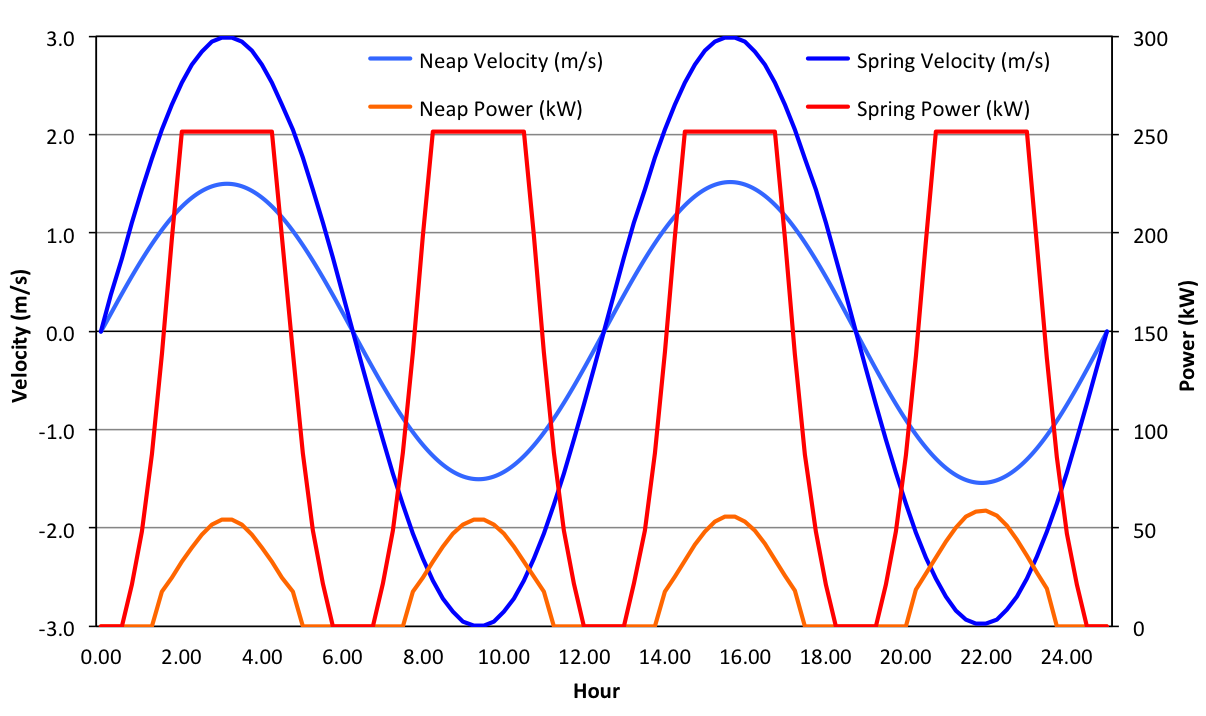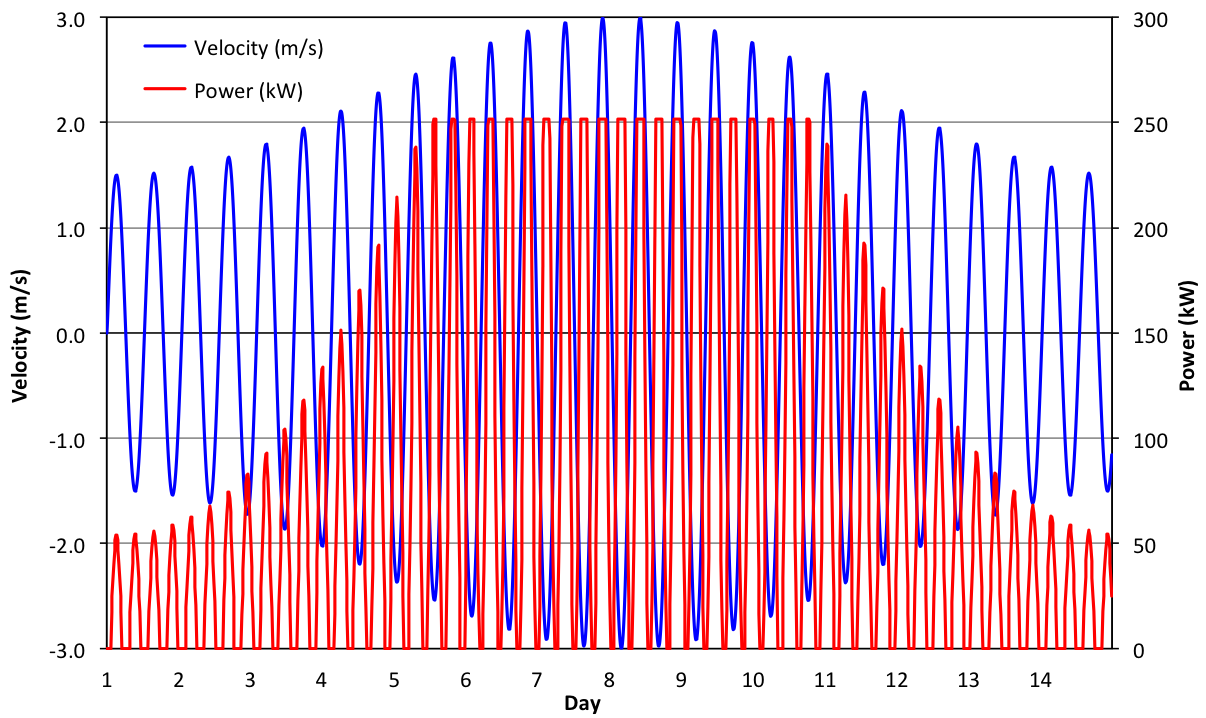ECONOMIC

## Energy Generation

In order to calculate the annual financial income from the turbine, the predicted annual energy generated was calculated as follows. Note that the energy output was calculated for a hypothetical site, with spring and neap tide current velocities of 3.0 m/s and 1.5 m/s respectively. However, these velocities are comparable with those experienced at the EMEC tidal test site (Fall of Warness), where the spring velocity is 3.5 m/s and the neap tide velocity is 1.4 m/s (Norris and Droniou, 2007).

### Assumptions

• Diameter = 10 m
• Power coefficient = 0.4
• Cut-in velocity = 1.0 m/s
• Rated velocity = 2.5 m/s
• Rated power = 250 kW
• Maximum velocity at spring tides = 3.0 m/s
• Maximum velocity at neap tides = 1.5 m/s
• Diurnal tidal period = 12.5 hours
• Bi-monthly neap-spring period = 14 days
• ### Diurnal Tidal Cycle

The chart below shows the power output from the turbine over two diurnal tidal cycles, at both spring tides and neap tides. During spring tides the power output reaches the rated power of 250 kW for several hours at a time. However, during neap tides the power output reaches a maximum of only 55 kW, or 22% of the rated power.### Bi-Monthly Tidal Cycle

The chart below shows the power output from the turbine over one bi-monthly tidal cycle. This cycle is repeated twenty-six times over the course of the year.### Total Annual Energy

The total annual energy output was calculated to be 701.5 MWh.